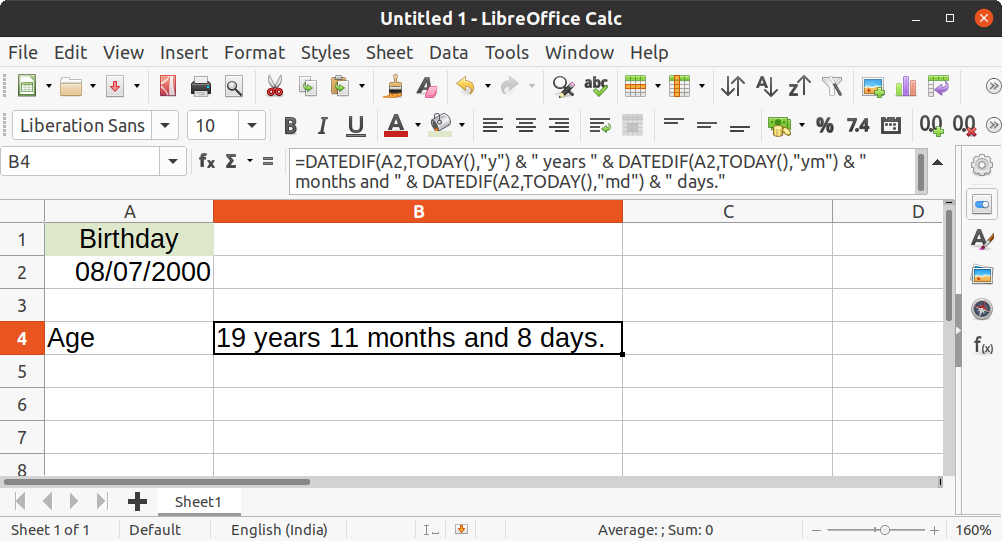# How to Calculate Date Difference in LibreOffice Calc

Having trouble with date calculations in LibreOffice Calc? In this guide, you will learn how to calculate the types of date difference in LibreOffice Calc.

## Calculate Date Difference

Its always better to use a function to calculate the date difference. `DATEDIF` the function does just that. This function returns the number of whole days, months, years between two given start and end dates.

#### Syntax

`=DATEDIF(Start date; End date; Interval)`
• Start date and End date are the date range for your calculation.
• Interval is a string and accepted values are below.

## Examples

#### Number of days between two dates

If you want to calculate the number of days between two dates, use the `DATEDIF` function with interval `"d"`.

`=DATEDIF(A2,B2,"d")`

#### Number of months between two dates

In the above example, replace the interval with `m` to find out the number of months between two dates.

`=DATEDIF(A2,B2,"m")`

#### Number of years between two dates

Likewise, use the interval value `y` to calculate the number of years between two dates.

`=DATEDIF(A2,B2,"y")`

#### Number of months ignoring years

If you want to calculate the number of months between two dates ignoring the years, then use `ym` in the interval parameter.

SEE ALSO:  Count Cells with Strings, Numbers Using COUNTIF Function - Calc

#### Number of days ignoring months

Similarly to calculate the number of days between two dates ignoring the months, then use `ym` in the interval parameter.

#### Number of days ignoring years

Likewise, if you want to calculate the number of days between two dates ignoring the years, then use `yd` in the interval parameter.

#### Age calculation using birthday

You can also calculate age using the birthday of a person using the below formula by calculating `DATEDIF` between birthday and today’s date (via `TODAY()` function).

## Usage Notes

If you do a `DATEDIF` between two dates where your end date is less than your start date then you would get `Err:502`. So, in those cases, you can use `=MONTH() `or `DAY() `function and do subtraction between them.

Reference

#### Looking for something else?

Read our complete tutorial index of Calc, Writer, Impress and Draw.## Related#### arindam

IT professional by profession and founder of libreofficehelp.com. Loving Linux and other technologies since 2002. I believe in open source and its philosophy. Follow me on Twitter or email me.

## One thought to “How to Calculate Date Difference in LibreOffice Calc”

1.Setiadjit Djoko Santoso DVM says:

How to link a cell from diffrence worksheet or file; Sometime it doesn’t working;
Thank your